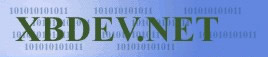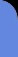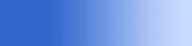Saturday July 4, 2020
 home | about | contact | DonationsThe Maths of 3D You can't have 3D without a little maths...

Sphere with OBB Collision Detection

by bkenwright@xbdev.net

Well I don't have the time to go into the full details, but its usually necessary to determine if a sphere-obb collision has occurred.  Its usually a lot simpler with AABB, but its not usually like this in the real 3D world, so this example shows the code working...its only very simplified, as I suppose it would be better to have the box rotating, and have it as a true 3d cube, but I'm keeping it as simple as possible.

 Downloadable ExecutableSource Code Snippet. // Given point p, return point q on (or in) OBB b, closest to p void ClosestPtInOBB(D3DXVECTOR3 p,   D3DXVECTOR3 bc,   D3DXVECTOR3* bu,   float* be,   D3DXVECTOR3 &q ) {        D3DXVECTOR3 d = p - bc;         // Start result at center of box; make steps from there        q = bc;        // For each OBB axis...        for (int i = 0; i < 3; i++)        {         // ...project d onto that axis to get the distance         // along the axis of d from the box center         float dist = D3DXVec3Dot(&d, &bu[i]);         // If distance farther than the box extents, clamp to the box         if (dist > be[i]) dist = be[i];         if (dist < -be[i]) dist = -be[i];         // Step that distance along the axis to get world coordinate         q += dist * bu[i];     } } // Returns true if sphere s intersects OBB b, false otherwise. // The point p on the OBB closest to the sphere center is also returned int SimpleSphereOBB_2D( D3DXVECTOR3 sc, float sr, D3DXVECTOR3 *boxCorners, D3DXVECTOR3 &p) { D3DXVECTOR3 bMin = D3DXVECTOR3(0,0,0); D3DXVECTOR3 bMax = D3DXVECTOR3(0,0,0); D3DXVECTOR3 obbCentre; int i = 0; bMin.x = boxCorners[i].x; bMin.y = boxCorners[i].y; bMin.z = boxCorners[i].z; bMax.x = boxCorners[i].x; bMax.y = boxCorners[i].y; bMax.z = boxCorners[i].z; for (i=0; i<4; i++) { bMin.x = min(bMin.x, boxCorners[i].x); bMin.y = min(bMin.y, boxCorners[i].y); bMin.z = min(bMin.z, boxCorners[i].z); bMax.x = max(bMax.x, boxCorners[i].x); bMax.y = max(bMax.y, boxCorners[i].y); bMax.z = max(bMax.z, boxCorners[i].z); } obbCentre = (bMax - bMin)/2.0f; float be; D3DXVECTOR3 bu; bu = boxCorners - boxCorners; be = D3DXVec3Length(&bu); D3DXVec3Normalize(&bu, &bu);     bu = boxCorners - boxCorners; be = D3DXVec3Length(&bu); D3DXVec3Normalize(&bu, &bu); bu = D3DXVECTOR3(0,0,0); be = 0.0f;     // Find point p on OBB closest to sphere center     ClosestPtPointOBB(sc,   obbCentre,   bu,   be,   p);     // Sphere and OBB intersect if the (squared) distance from sphere     // center to point p is less than the (squared) sphere radius     D3DXVECTOR3 v = p - sc;     return D3DXVec3Dot(&v, &v) <= sr * sr; }

 Visitor: 9534626  { 209.237.238.175 } Copyright (c) 2002-2020 xbdev.net - All rights reserved. Designated tutorial and software are the property of their respective owners.